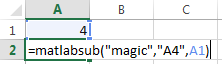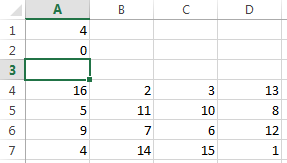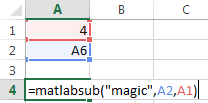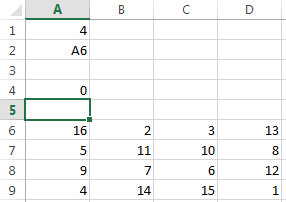# matlabsub

Evaluate MATLAB command given Microsoft Excel data and designate output location

## Syntax

``= matlabsub(command,result,inputs)``

## Description

example

````= matlabsub(command,result,inputs)` specifies the MATLAB® command for evaluation in the MATLAB workspace, given the input data `inputs`. The function returns the MATLAB output into the worksheet cell specified by `result`. Use this syntax when working in a worksheet cell.```

## Examples

collapse all

Enter the number four in cell `A1`.

Enter this text into cell `A2`. Specify the function `magic` as the command. Return the fourth-order magic square into the range of cells starting in cell `A4`. Reference cell `A1` as the input argument for the `magic` function.

`=matlabsub("magic","A4",A1)`The fourth-order magic square displays in the range of cells from `A4` through `D7`.Enter the number four in cell `A1`. Enter a target cell reference to cell `A6` in cell `A2`.

Enter this text into cell `A4`. Specify the function `magic` as the command. Return the fourth-order magic square into the range of cells starting in cell `A6` by entering the cell reference `A2`. Reference cell `A1` as the input argument for the `magic` function.

`=matlabsub("magic",A2,A1)`The fourth-order magic square displays in the range of cells from `A6` through `D9`.## Input Arguments

collapse all

MATLAB command to evaluate, specified as a string. Enclose the string in double quotes. Or, enter the string in a cell without quotes and enter the corresponding cell reference without quotes as the input argument.

Example: `"sum"`

Example: `A1`

MATLAB result, specified as an Excel cell reference or range name that designates the location where to display the result of the MATLAB command. To return the result in a specific cell, specify the cell reference enclosed in quotes. To denote a worksheet cell address or range name that contains a cell reference to another cell, specify the cell reference without quotes.

Example: `"A3"`

Example: `D6`

MATLAB command input arguments, specified as an Excel cell reference or a reference to a range of cells. To specify multiple input arguments for a function, separate the cell references with commas.

Example: `B1:B10`

Example: `A1:C1,A3`

## Tips

• To return an array of data to the Microsoft® Excel Visual Basic® for Applications (VBA) workspace, see `MLEvalString` and `MLGetVar`.

• `result` must not include the cell that contains `matlabsub`. Do not overwrite the function itself.

• Ensure that there is enough room in the worksheet for writing matrix contents. If there is insufficient room, the function generates a fatal error.

• If `matlabsub` fails, a standard Spreadsheet Link™ error displays by default; for example, `#COMMAND`. To return MATLAB errors, use `MLShowMatlabErrors`.